# Bessel system

(diff) ← Older revision | Latest revision (diff) | Newer revision → (diff)

A concept in the theory of orthogonal systems. Letand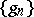be two complete systems of functions in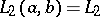(i.e. measurable functions that are square-integrable on the segment), forming a biorthogonal system of functions. The system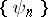is said to be a Bessel system if, for any function, the seriesis convergent; here,are the coefficients of the expansion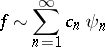of the functionwith respect to the system. For a systemto be a Bessel system it is necessary and sufficient that it be possible to define a bounded linear operatoron the space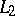such that the systemdefined by the equation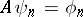() is a complete orthonormal system. If the systemis a Bessel system, there exists a constantsuch that for any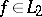How to Cite This Entry:
Bessel system. Encyclopedia of Mathematics. URL: http://encyclopediaofmath.org/index.php?title=Bessel_system&oldid=13693
This article was adapted from an original article by P.I. Lizorkin (originator), which appeared in Encyclopedia of Mathematics - ISBN 1402006098. See original article